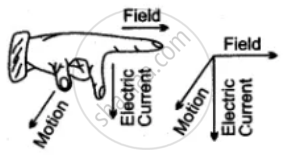# State Fleming'S Left Hand Rule. - Science

Definition
Short Note

State Fleming's left hand rule.

Name and state the law used to find the direction of force on a current carrying conductor kept in a magnetic field.

#### Solution 1According to this rule, stretch the thumb, forefinger and middle finger of your left hand such that they are mutually perpendicular. If the first finger points in the direction of magnetic field and the second finger in the direction of the current, then the thumb will point in the direction of motion of the conductor or the force acting on the conductor.

#### Solution 2

Fleming's left hand rule can be stated as: Stretch the forefinger, the middle finger and the thumb of the right hand, such that they are mutually perpendicular to each other. If the forefinger indicates the direction of the magnetic field, the middle finger indicates the direction of current in the conductor then thumb indicates the direction of force exerted on the conductor.

Concept: Force on a Current Carrying Conductor in a Magnetic Field
Is there an error in this question or solution?

#### APPEARS IN

Frank ICSE Class 10 Physics Part 2
Chapter 4 Current Electricity
Exercise 4.3 | Q 18 | Page 206
ICSE Class 10 Physics
Chapter 9 Magnetic Effect of Current
ICSE Class 10 Physics
Chapter 9 Magnetic Effect of Current
Figure Based Short Answers | Q 6